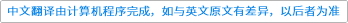International Journal of Mathematics ( IF 0.604 ) Pub Date : 2021-04-10 , DOI: 10.1142/s0129167x21500361
Sourav Pal, Samriddho Roy

We find new characterizations for the points in the symmetrized polydisc$𝔾n$, a family of domains associated with the spectral interpolation, defined by $𝔾n:=∑1≤i≤nzi,∑1≤i We introduce a new family of domains which we call the extended symmetrized polydisc$𝔾̃n$, and define in the following way: $𝔾̃n:=(y1,…,yn−1,q)∈ℂn:q∈𝔻,yj=βj+β̄n−jq,βj∈ℂand$$|βj|+|βn−j| We show that $𝔾n=𝔾̃n$ for $n=1,2$ and that $𝔾n⊊𝔾̃n$ for $n≥3$. We first obtain a variety of characterizations for the points in $𝔾̃n$ and we apply these necessary and sufficient conditions to produce an analogous set of characterizations for the points in $𝔾n$. Also, we obtain similar characterizations for the points in $Γn∖𝔾n$, where $Γn=𝔾n¯$. A set of $n−1$ fractional linear transformations plays central role in the entire program. We also show that for $n≥2$, $𝔾̃n$ is nonconvex but polynomially convex and is starlike about the origin but not circled.down
wechat
bug